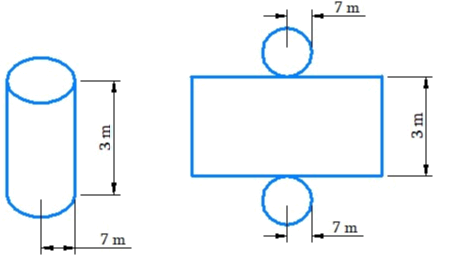# Ex.11.3 Q7 Mensuration Solution - NCERT Maths Class 8

Go back to  'Ex.11.3'

## Question

A closed cylindrical tank of radius $$7 \,\rm{m}$$ and height $$3\,\rm{ m}$$ is made from a sheet of metal. How much sheet of metal is required?

Video Solution
Mensuration
Ex 11.3 | Question 7

## Text Solution

What is Known?

Radius and height of cylindrical tank.

What is Unknown?

Metal sheet required for cylindrical tank.

Reasoning:The length of rectangular sheet will be equal to the circumference of the circle. Visually total surface of the cylinder will be equal to sum of lateral surface area and area of two circles.

Steps:

Height of the cylindrical tank $$(h) = 3\,\rm{cm}$$

Radius of the cylindrical $$(r) = 7\,\rm{cm}$$

Lateral surface area of the cylinder

\begin{align}&=2\pi rh \\&= 2 \times \frac{{22}}{7} \times 7 \times 3 \\&= 132\, \rm{m^2} \qquad \dots (\rm{1}) \end{align}

Area of circle

\begin{align}&= \pi {r^2} \\&= 2 \times \frac{{22}}{7} \times 7{\rm{m}} \times 7{\rm{m}} \\&=154\,{{\rm{m}}^2} \qquad \dots (\rm{2}) \end{align}

Total surface area of cylinder = Lateral surface area of the cylinder + Area of two circles
\begin{align}& = 2\pi rh + 2\pi {r^2}\\& = 132\, \rm{m^2} + 308 \,\rm{m^2}\\& \;\text{(putting values from (1) and (2))}\\ &= 440 \,\rm{m^2}\end{align}

Thus, $$440\,\rm{m^2}$$ sheet is required.

Learn from the best math teachers and top your exams

• Live one on one classroom and doubt clearing
• Practice worksheets in and after class for conceptual clarity
• Personalized curriculum to keep up with school